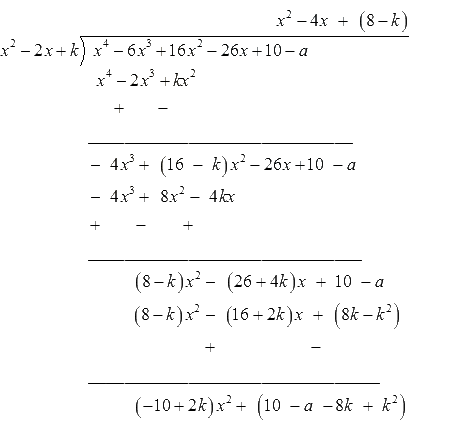# Ex.2.4 Q5 Polynomials Solution - NCERT Maths Class 10

## Question

If the polynomial $${x^4}-{{ }}6{x^3} + {{ }}16{x^2}-{{ }}25x + {{ }}10$$ is divided by another polynomial $${x^2}-{{ }}2x + k,$$ the remainder comes out to be $$x + a,$$ find $$k$$ and $$a.$$

Video Solution
Polynomials
Ex 2.4 | Question 5

## Text Solution

What is known?

If the polynomial $${x^4}-6{x^3} + {{ }}16{x^2}-25x + {{ }}10$$ is divided by another polynomial $${x^2}-2x + k,$$ the remainder comes out to be $$x+a.$$

What is unknown?

Value of $$k$$ and $$a.$$

Reasoning:

This question is straight forward, use division algorithm and put the known values of dividend, divisor and remainder in the division algorithm and get the Quotient. Now you will get the remainder, but we have already subtracted remainder that means ideally the remainder should be equal to zero. Now, on equating remainder with zero, you will get the value of $$k$$ and $$a.$$

Steps:

By Division algorithm,

Dividend $$=$$ Divisior $$\times$$ Quotient  $$+$$  Remainder

\begin{align}\begin{bmatrix}{x^4}\! -\! 6{x^3} \!+ \!16{x^2} \\\! -\! 25x + 10\end{bmatrix} &\!=\! \!\begin{bmatrix}\left( {{x^2} \!- \!2x \!+\! k} \right) \\ \! \times \! Q \!+\! \left( {x \!+\! {{a}}} \right)\end{bmatrix}\\ \begin{bmatrix}{x^4} \!-\! 6{x^3} \!+ \!16{x^2} \!- \!\\26x\! +\! 10 \!-\! a \end{bmatrix}&\!=\! \!{\left( {{x^2}\! - \!2x \!+ \!k} \right) \!\times\! Q}\\{\frac{\begin{bmatrix}{x^4} - 6{x^3} - 26x \\+ 10 - a\end{bmatrix}}{{\left( {{x^2} - 2x + k} \right)}}} &= Q\end{align}On comparing

\begin{align} \left( -10+2k \right)&=0 \\ 2k&=10 \\k & = 5 \\\end{align}

\begin{align}10 - a - 8k + {k^2}& = 0\\10 - a - 8(5) + {{(5)}^2} &= 0\\10 - a - 40 + 25 &= 0\\- 30 + 25 - a &= 0\\- 5 - a &= 0\\a &= - 5\end{align}

Hence \begin{align} k = 5, \;a = - 5 \end{align}

Learn from the best math teachers and top your exams

• Live one on one classroom and doubt clearing
• Practice worksheets in and after class for conceptual clarity
• Personalized curriculum to keep up with school